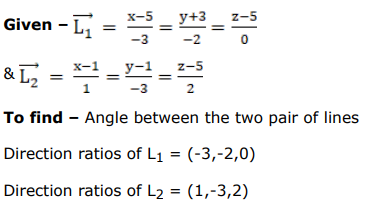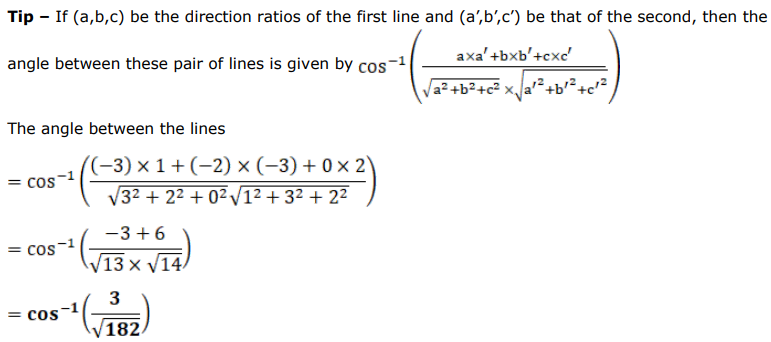# Find the angle between each of the following pairs of lines:

Question:

Find the angle between each of the following pairs of lines:

$\frac{5-x}{3}=\frac{y+3}{-2}, z=5$ and $\frac{x-1}{1}=\frac{1-y}{3}=\frac{z-5}{2}$

Solution: